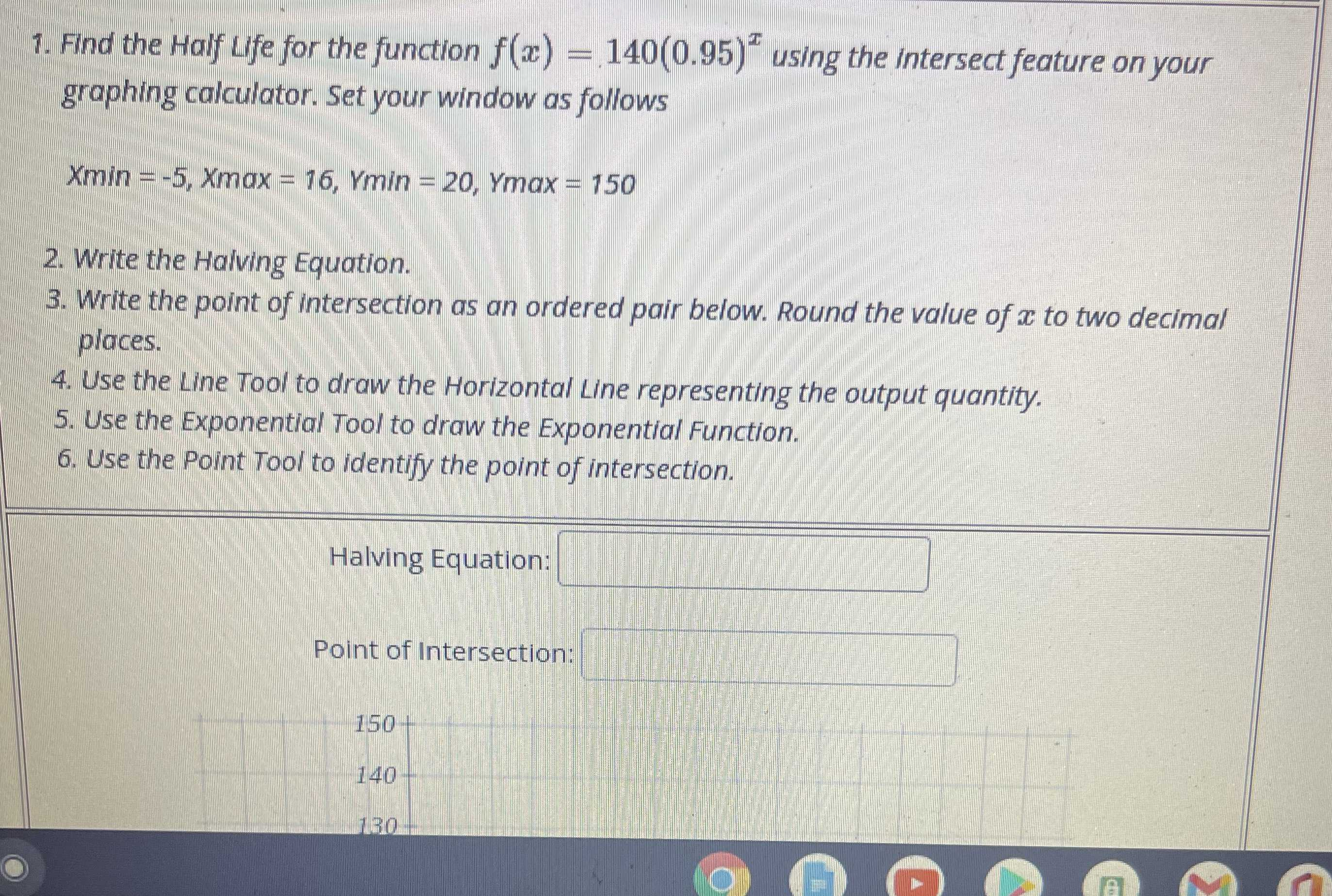### ¿Todavía tienes preguntas de matemáticas?

Pregunte a nuestros tutores expertos
Algebra
Pregunta1. Find the Half Life for the function $$f ( x ) = 140 ( 0.95 ) ^ { x }$$ using the intersect feature on your graphing calculator. Set your window as follows. $$x \min = - 5 , x \max = 16 , Y \min = 20 , Y \max = 150$$

2. Write the Haiving Equation. 3. Write the point of intersection as an ordered pair below. Round the value of $$x$$ to two decimal places.